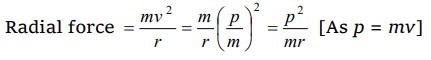## Motion in a Plane Questions and Answers Part-4

1. A mass of 100 gm is tied to one end of a string 2 m long. The body is revolving in a horizontal circle making a maximum of 200 revolutions per min. The other end of the string is fixed at the centre of the circle of revolution. The maximum tension that the string can bear is (approximately)
a) 8.76 N
b) 8.94 N
c) 89.42 N
d) 87.64 N

Explanation:2. A road is 10 m wide. Its radius of curvature is 50 m. The outer edge is above the lower edge by a distance of 1.5 m. This road is most suited for the velocity
a) 2.5 m/sec
b) 4.5 m/sec
c) 6.5 m/sec
d) 8.5 m/sec

Explanation: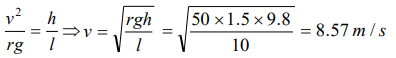3. Certain neutron stars are believed to be rotating at about 1rev / sec . If such a star has a radius of 20 km, the acceleration of an object on the equator of the star will be
a) $20\times10^{8}m\diagup sec^{2}$
b) $8\times10^{5}m\diagup sec^{2}$
c) $120\times10^{5}m\diagup sec^{2}$
d) $4\times10^{8}m\diagup sec^{2}$

Explanation: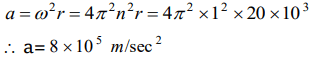4. A particle revolves round a circular path. The acceleration of the particle is
a) Along the circumference of the circle
b) Along the tangent
d) Zero

5. The length of second's hand in a watch is 1 cm. The change in velocity of its tip in 15 seconds is
a) Zero
b) $\frac{\pi}{30\sqrt{2}}cm\diagup sec$
c) $\frac{\pi}{30}cm\diagup sec$
d) $\frac{\pi\sqrt{2}}{30}cm\diagup sec$

Explanation: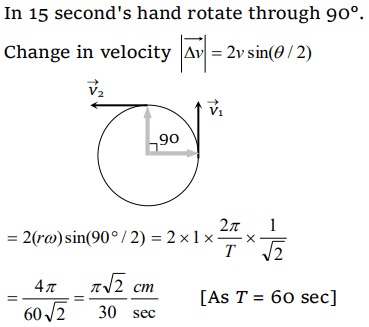6. A particle moves in a circle of radius 25 cm at two revolutions per second. The acceleration of the particle in $m\diagup sec^{2}$  is
a) $\pi^{2}$
b) $8\pi^{2}$
c) $4\pi^{2}$
d) $2\pi^{2}$

Explanation: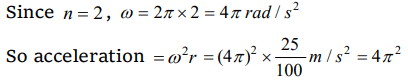7. An electric fan has blades of length 30 cm as measured from the axis of rotation. If the fan is rotating at 1200 r.p.m. The acceleration of a point on the tip of the blade is about
a) $1600 m\diagup sec^{2}$
b) $4740 m\diagup sec^{2}$
c) $2370 m\diagup sec^{2}$
d) $5055 m\diagup sec^{2}$

Explanation:8. The force required to keep a body in uniform circular motion is
a) Centripetal force
b) Centrifugal force
c) Resistance
d) None of the above

Explanation: Centripetal force

9. Cream gets separated out of milk when it is churned, it is due to
a) Gravitational force
b) Centripetal force
c) Centrifugal force
d) Frictional force

b) $\frac{rm}{p}$
c) $\frac{mp^{2}}{r}$
d) $\frac{p^{2}}{rm}$